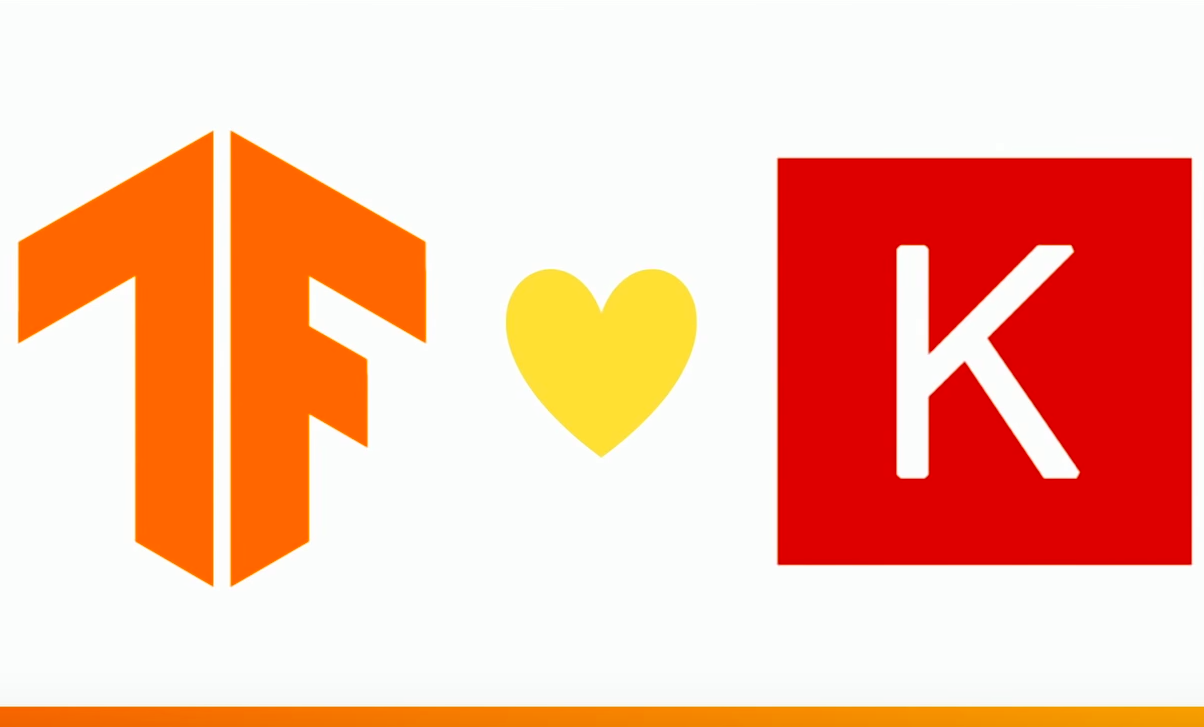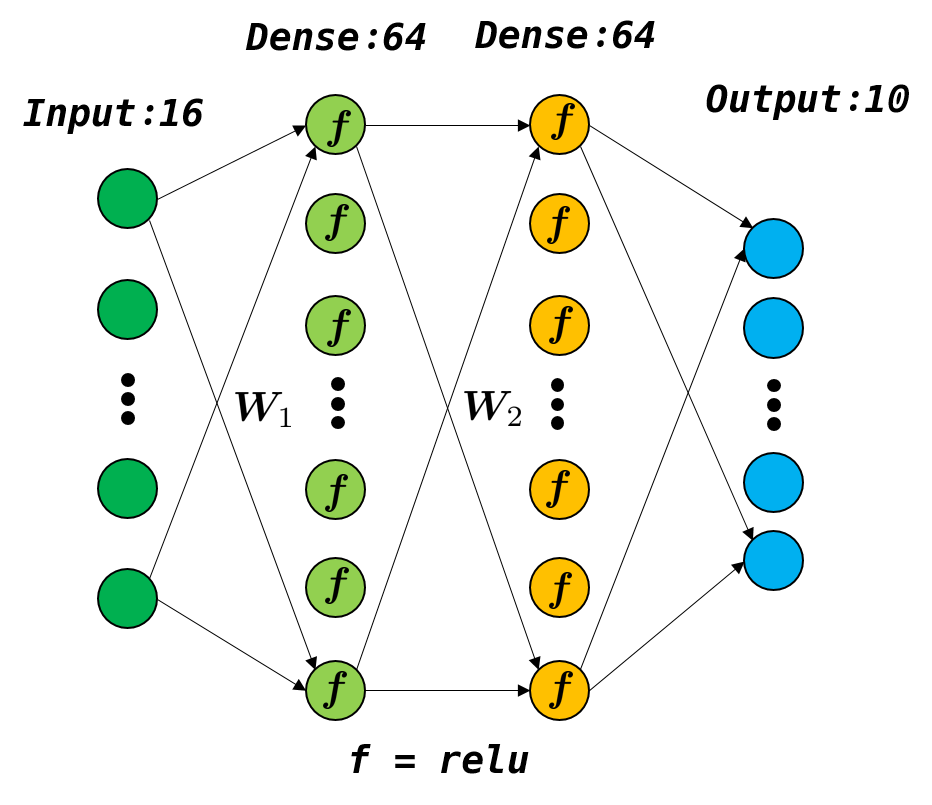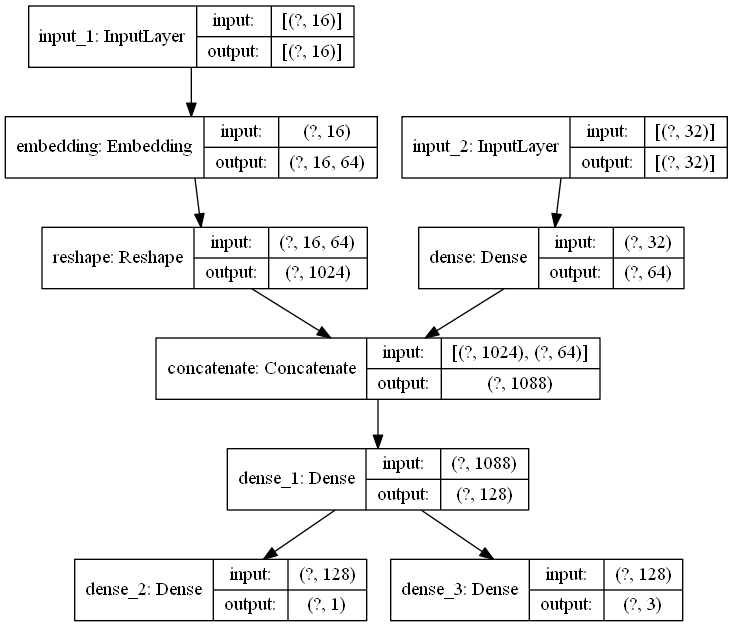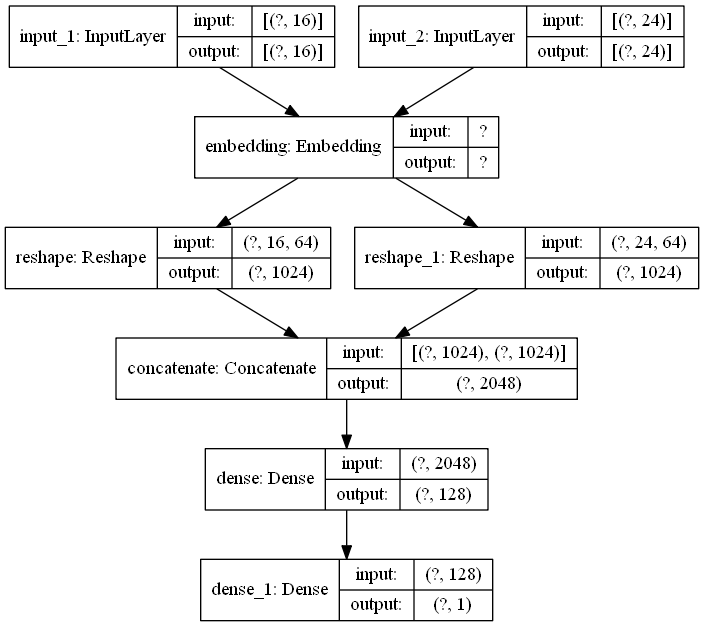# TensorFlow 篇 | TensorFlow 2.x 基于 Keras 的模型构建## Keras 介绍

1. Keras 是一个用 Python 编写的高级神经网络 API ，它是一个独立的库，能够以 TensorFlowCNTK 或者 Theano 作为后端运行。 TensorFlow1.0 版本开始尝试与 Keras 做集成，到 2.0 版发布后更是深度集成了 Keras ，并紧密依赖 tf.keras 作为其中央高级 API ，官方亦高度推荐使用 keras API 来完成深度模型的构建。

2. tf.keras 具有三个关键优势：

1. 对小白用户友好： Keras 具有简单且一致的接口，并对用户产生的错误有明确可行的建议去修正。 TensorFlow 2.0 之前的版本，由于其代码编写复杂， API 接口混乱而且各个版本之间兼容性较差，受到广泛的批评，使用 Keras 进行统一化之后，会大大减少开发人员的工作量。

2. 模块化且可组合： Keras 模型通过可构建的模块连接在一起，没有任何限制，模型结构清晰，代码容易阅读。

3. 便于扩展：当编写新的自定义模块时，可以非常方便的基于已有的接口进行扩展。

3. Keras 使得 TensorFlow 更易于使用，而且不用损失其灵活性和性能。

## Keras 模型构建

TensorFlow 2.x 版本中，可以使用三种方式来构建 Keras 模型，分别是 Sequential函数式 (Functional) API 以及自定义模型 (Subclassed)。下面就分别介绍下这三种构建方式。

### Sequential Model1. Keras 中，通常是将多个层 (layer) 组装起来形成一个模型 (model)，最常见的一种方式就是层的堆叠，可以使用 tf.keras.Sequential 来轻松实现。以上图中所示模型为例，其代码实现如下：

import tensorflow as tf
from tensorflow.keras import layers

model = tf.keras.Sequential()
# Adds a densely-connected layer with 64 units to the model:
# This is identical to the following:
# Add an output layer with 10 output units:
# model.build((None, 16))
print(model.weights)

2. 注意对于 Sequential 添加的第一层，可以包含一个 input_shapeinput_dimbatch_input_shape 参数来指定输入数据的维度，详见注释部分。当指定了 input_shape 等参数后，每次 add 新的层，模型都在持续不断地创建过程中，也就说此时模型中各层的权重矩阵已经被初始化了，可以通过调用 model.weights 来打印模型的权重信息。

3. 当然，第一层也可以不包含输入数据的维度信息，称之为延迟创建模式，也就是说此时模型还未真正创建，权重矩阵也不存在。可以通过调用 model.build(batch_input_shape) 方法手动创建模型。如果未手动创建，那么只有当调用 fit 或者其他训练和评估方法时，模型才会被创建，权重矩阵才会被初始化，此时模型会根据输入的数据来自动推断其维度信息。

4. input_shape 中没有指定 batch 的大小而将其设置为 None ，是因为在训练与评估时所采用的 batch 大小可能不一致。如果设为定值，在训练或评估时会产生错误，而这样设置后，可以由模型自动推断 batch 大小并进行计算，鲁棒性更强。

5. 除了这种顺序性的添加 (add) 外，还可以通过将 layers 以参数的形式传递给 Sequential 来构建模型。示例代码如下所示：

import tensorflow as tf
from tensorflow.keras import layers

model = tf.keras.Sequential([
layers.Dense(64, activation='relu', input_shape=(16, )),
layers.Dense(64, activation='relu'),
layers.Dense(10)
])
# model.build((None, 16))
print(model.weights)


### 函数式 API

1. Keras函数式 API 是比 Sequential 更为灵活的创建模型的方式。它可以处理具有非线性拓扑结构的模型、具有共享层 (layers) 的模型以及多输入输出的模型。深度学习的模型通常是由层 (layers) 组成的有向无环图，而函数式 API 就是构建这种图的一种有效方式。

2. Sequential Model 一节中提到的模型为例，使用函数式 API 实现的方式如下所示：

from tensorflow import keras
from tensorflow.keras import layers

inputs = keras.Input(shape=(16, ))
dense = layers.Dense(64, activation='relu')
x = dense(inputs)
x = layers.Dense(64, activation='relu')(x)
outputs = layers.Dense(10)(x)
model = keras.Model(inputs=inputs, outputs=outputs, name='model')
model.summary()

3. 与使用 Sequential 方法构建模型的不同之处在于，函数式 API 通过 keras.Input 指定了输入 inputs 并通过函数调用的方式生成了输出 outputs ，最后使用 keras.Model 方法构建了整个模型。

4. 为什么叫函数式 API ，从代码中可以看到，可以像函数调用一样来使用各种层 (layers)，比如定义好了 dense 层，可以直接将 inputs 作为 dense 的输入而得到一个输出 x ，然后又将 x 作为下一层的输入，最后的函数返回值就是整个模型的输出。

5. 函数式 API 可以将同一个层 (layers) 作为多个模型的组成部分，示例代码如下所示：

from tensorflow import keras
from tensorflow.keras import layers

encoder_input = keras.Input(shape=(16, ), name='encoder_input')
x = layers.Dense(32, activation='relu')(encoder_input)
x = layers.Dense(64, activation='relu')(x)
encoder_output = layers.Dense(128, activation='relu')(x)

encoder = keras.Model(encoder_input, encoder_output, name='encoder')
encoder.summary()

x = layers.Dense(64, activation='relu')(encoder_output)
x = layers.Dense(32, activation='relu')(x)
decoder_output = layers.Dense(16, activation='relu')(x)

autoencoder = keras.Model(encoder_input, decoder_output, name='autoencoder')
autoencoder.summary()


代码中包含了两个模型，一个编码器 (encoder) 和一个自编码器 (autoencoder)，可以看到两个模型共用了 encoder_out 层，当然也包括了 encoder_out 层之前的所有层。

6. 函数式 API 生成的所有模型 (models) 都可以像层 (layers) 一样被调用。还以自编码器 (autoencoder) 为例，现在将它分成编码器 (encoder) 和解码器 (decoder) 两部分，然后用 encoderdecoder 生成 autoencoder ，代码如下：

from tensorflow import keras
from tensorflow.keras import layers

encoder_input = keras.Input(shape=(16, ), name='encoder_input')
x = layers.Dense(32, activation='relu')(encoder_input)
x = layers.Dense(64, activation='relu')(x)
encoder_output = layers.Dense(128, activation='relu')(x)

encoder = keras.Model(encoder_input, encoder_output, name='encoder')
encoder.summary()

decoder_input = keras.Input(shape=(128, ), name='decoder_input')
x = layers.Dense(64, activation='relu')(decoder_input)
x = layers.Dense(32, activation='relu')(x)
decoder_output = layers.Dense(16, activation='relu')(x)

decoder = keras.Model(decoder_input, decoder_output, name='decoder')
decoder.summary()

autoencoder_input = keras.Input(shape=(16), name='autoencoder_input')
encoded = encoder(autoencoder_input)
autoencoder_output = decoder(encoded)
autoencoder = keras.Model(
autoencoder_input,
autoencoder_output,
name='autoencoder',
)
autoencoder.summary()


代码中首先生成了两个模型 encoderdecoder ，然后在生成 autoencoder 模型时，使用了模型函数调用的方式，直接将 autoencoder_inputencoded 分别作为 encoderdecoder 两个模型的输入，并最终得到 autoencoder 模型。

7. 函数式 API 可以很容易处理多输入和多输出的模型，这是 Sequential API 无法实现的。比如我们的模型输入有一部分是类别型特征 ，一般需要经过 Embedding 处理，还有一部分是数值型特征，一般无需特殊处理，显然无法将这两种特征直接合并作为单一输入共同处理，此时就会用到多输入。而有时我们希望模型返回多个输出，以供后续的计算使用，此时就会用到多输出模型。多输入与多输出模型的示例代码如下所示：

from tensorflow import keras
from tensorflow.keras import layers

categorical_input = keras.Input(shape=(16, ))
numeric_input = keras.Input(shape=(32, ))
categorical_features = layers.Embedding(
input_dim=100,
output_dim=64,
input_length=16,
)(categorical_input)
categorical_features = layers.Reshape([16 * 64])(categorical_features)
numeric_features = layers.Dense(64, activation='relu')(numeric_input)
x = layers.Concatenate(axis=-1)([categorical_features, numeric_features])
x = layers.Dense(128, activation='relu')(x)

binary_pred = layers.Dense(1, activation='sigmoid')(x)
categorical_pred = layers.Dense(3, activation='softmax')(x)

model = keras.Model(
inputs=[categorical_input, numeric_input],
outputs=[binary_pred, categorical_pred],
)
model.summary()


代码中有两个输入 categorical_inputnumeric_input ，经过不同的处理层后，二者通过 Concatenate 结合到一起，最后又经过不同的处理层得到了两个输出 binary_predcategorical_pred 。该模型的结构图如下图所示：8. 函数式 API 另一个好的用法是模型的层共享，也就是在一个模型中，层被多次重复使用，它从不同的输入学习不同的特征。一种常见的共享层是嵌入层 (Embedding)，代码如下：

from tensorflow import keras
from tensorflow.keras import layers

categorical_input_one = keras.Input(shape=(16, ))
categorical_input_two = keras.Input(shape=(24, ))

shared_embedding = layers.Embedding(100, 64)

categorical_features_one = shared_embedding(categorical_input_one)
categorical_features_two = shared_embedding(categorical_input_two)

categorical_features_one = layers.Reshape([16 * 64])(categorical_features_one)
categorical_features_two = layers.Reshape([16 * 64])(categorical_features_two)

x = layers.Concatenate(axis=-1)([
categorical_features_one,
categorical_features_two,
])
x = layers.Dense(128, activation='relu')(x)
outputs = layers.Dense(1, activation='sigmoid')(x)

model = keras.Model(
inputs=[categorical_input_one, categorical_input_two],
outputs=outputs,
)
model.summary()


代码中有两个输入 categorical_input_onecategorical_input_two ，它们共享了一个 Embeddingshared_embedding 。该模型的结构图如下图所示：### 自定义 Keras 层和模型

1. tf.keras 模块下包含了许多内置的层 (layers)，比如上面我们用到的 DenseEmbeddingReshape 等。有时我们会发现这些内置的层并不能满足我们的需求，此时可以很方便创建自定义的层来进行扩展。自定义的层通过继承 tf.keras.Layer 类来实现，且该子类要实现父类的 buildcall 方法。对于内置的 Dense 层，使用自定义层来实现的话，其代码如下所示：

import tensorflow as tf
from tensorflow import keras
from tensorflow.keras import layers

class CustomDense(layers.Layer):
def __init__(self, units=32):
super().__init__()
self.units = units

def build(self, input_shape):
shape=(input_shape[-1], self.units),
initializer='random_normal',
trainable=True,
)
shape=(self.units, ),
initializer='random_normal',
trainable=True,
)

def call(self, inputs):
return tf.matmul(inputs, self.w) + self.b

def get_config(self):
return {'units': self.units}

@classmethod
def from_config(cls, config):
return cls(**config)

inputs = keras.Input((4, ))
layer = CustomDense(10)
outputs = layer(inputs)

model = keras.Model(inputs, outputs)
model.summary()

# layer recreate
config = layer.get_config()
new_layer = CustomDense.from_config(config)
new_outputs = new_layer(inputs)
print(new_layer.weights)
print(new_layer.non_trainable_weights)
print(new_layer.trainable_weights)

# model recreate
config = model.get_config()
new_model = keras.Model.from_config(
config,
custom_objects={'CustomDense': CustomDense},
)
new_model.summary()

1. 其中 __init__ 方法用来初始化一些构建该层所需的基本参数， build 方法用来创建该层所需的权重矩阵 w 和偏差矩阵 bcall 方法则是层构建的真正执行者，它将输入转为输出并返回。其实权重矩阵等的创建也可以在 __init__ 方法中完成，但是在很多情况下，我们不能提前预知输入数据的维度，需要在实例化层的某个时间点来延迟创建权重矩阵，因此需要在 build 方法中根据输入数据的维度信息 input_shape 来动态创建权重矩阵。

2. 以上三个方法的调用顺序为 __init__buildcall ，其中 __init__ 在实例化层时即被调用，而 buildcall 是在确定了输入后才被调用。其实 Layer 类中有一个内置方法 __call__ ，在层构建时首先会调用该方法，而在方法内部会调用 buildcall ，并且只有第一次调用 __call__ 时才会触发 build ，也就是说 build 中的变量只能被创建一次，而 call 是可以被调用多次的，比如训练，评估时都会被调用。

3. 如果需要对该层提供序列化的支持，则需要实现一个 get_config 方法来以字典的形式返回该层实例的构造函数参数。在给定 config 的字典后，可以通过调用该层的类方法 (classmethod) from_config 来重新创建该层， from_config 的默认实现如代码所示，层的重新创建见 layer recreate 代码部分，当然也可以重写 from_config 类方法来提供新的创建方式。而重新创建新模型 (model) 的代码与 layer 重建的代码有所不同，它需要借助于 keras.Model.from_config 方法来完成构建，详见 model recreate 代码部分。

2. 自定义的层是可以递归组合的，也就是说一个层可以作为另一个层的属性。一般推荐在 __init__ 方法中创建子层，因为子层自己的 build 方法会在外层 build 调用时被触发而去执行权重矩阵的构建任务，无需在父层中显示创建。还以 Sequential Model 一节提到的模型为例作为说明，代码如下：

from tensorflow import keras
from tensorflow.keras import layers

class MLP(layers.Layer):
def __init__(self):
super().__init__()
self.dense_1 = layers.Dense(64, activation='relu')
self.dense_2 = layers.Dense(64, activation='relu')
self.dense_3 = layers.Dense(10)

def call(self, inputs):
x = self.dense_1(inputs)
x = self.dense_2(x)
x = self.dense_3(x)
return x

inputs = keras.Input((16, ))
mlp = MLP()

y = mlp(inputs)
print('weights:', len(mlp.weights))
print('trainable weights:', len(mlp.trainable_weights))


从代码中可以看到，我们将三个 Dense 层作为 MLP 的子层，然后利用它们来完成 MLP 的构建，可以达到与 Sequential Model 中一样的效果，而且所有子层的权重矩阵都会作为新层的权重矩阵而存在。

3. 层 (layers) 在构建的过程中，会去递归地收集在此创建过程中生成的损失 (losses)。在重写 call 方法时，可通过调用 add_loss 方法来增加自定义的损失。层的所有损失中也包括其子层的损失，而且它们都可以通过 layer.losses 属性来进行获取，该属性是一个列表 (list)，需要注意的是正则项的损失会自动包含在内。示例代码如下所示：

import tensorflow as tf
from tensorflow import keras
from tensorflow.keras import layers

class CustomLayer(layers.Layer):
def __init__(self, rate=1e-2, l2_rate=1e-3):
super().__init__()
self.rate = rate
self.l2_rate = l2_rate
self.dense = layers.Dense(
units=32,
kernel_regularizer=keras.regularizers.l2(self.l2_rate),
)

def call(self, inputs):
return self.dense(inputs)

inputs = keras.Input((16, ))
layer = CustomLayer()
x = layer(inputs)
print(layer.losses)

4. 层或模型的 call 方法预置有一个 training 参数，它是一个 bool 类型的变量，表示是否处于训练状态，它会根据调用的方法来设置值，训练时为 True ， 评估时为 False 。因为有一些层像 BatchNormalizationDropout 一般只会用在训练过程中，而在评估和预测的过程中一般是不会使用的，所以可以通过该参数来控制模型在不同状态下所执行的不同计算过程。

5. 自定义模型与自定义层的实现方式比较相似，不过模型需要继承自 tf.keras.ModelModel 类的有些 API 是与 Layer 类相同的，比如自定义模型也要实现 __init__buildcall 方法。不过两者也有不同之处，首先 Model 具有训练，评估以及预测接口，其次它可以通过 model.layers 查看所有内置层的信息，另外 Model 类还提供了模型保存和序列化的接口。以 AutoEncoder 为例，一个完整的自定义模型的示例代码如下所示：

from tensorflow import keras
from tensorflow.keras import layers

class Encoder(layers.Layer):
def __init__(self, l2_rate=1e-3):
super().__init__()
self.l2_rate = l2_rate

def build(self, input_shape):
self.dense1 = layers.Dense(
units=32,
activation='relu',
kernel_regularizer=keras.regularizers.l2(self.l2_rate),
)
self.dense2 = layers.Dense(
units=64,
activation='relu',
kernel_regularizer=keras.regularizers.l2(self.l2_rate),
)
self.dense3 = layers.Dense(
units=128,
activation='relu',
kernel_regularizer=keras.regularizers.l2(self.l2_rate),
)

def call(self, inputs):
x = self.dense1(inputs)
x = self.dense2(x)
x = self.dense3(x)
return x

class Decoder(layers.Layer):
def __init__(self, l2_rate=1e-3):
super().__init__()
self.l2_rate = l2_rate

def build(self, input_shape):
self.dense1 = layers.Dense(
units=64,
activation='relu',
kernel_regularizer=keras.regularizers.l2(self.l2_rate),
)
self.dense2 = layers.Dense(
units=32,
activation='relu',
kernel_regularizer=keras.regularizers.l2(self.l2_rate),
)
self.dense3 = layers.Dense(
units=16,
activation='relu',
kernel_regularizer=keras.regularizers.l2(self.l2_rate),
)

def call(self, inputs):
x = self.dense1(inputs)
x = self.dense2(x)
x = self.dense3(x)
return x

class AutoEncoder(keras.Model):
def __init__(self):
super().__init__()
self.encoder = Encoder()
self.decoder = Decoder()

def call(self, inputs):
x = self.encoder(inputs)
x = self.decoder(x)
return x

model = AutoEncoder()
model.build((None, 16))
model.summary()
print(model.layers)
print(model.weights)


上述代码实现了一个 AutoEncoder Model 类，它由两层组成，分别为 EncoderDecoder ，而这两层也是自定义的。通过调用 model.weights 可以查看该模型所有的权重信息，当然这里包含子层中的所有权重信息。

6. 对于自定义的层或模型，在调用其 summary, weights, variables, trainable_weightslosses 等方法或属性时，要先确保层或模型已经被创建，不然可能报错或返回为空，在模型调试时要注意这一点。

### 配置层 (layer)

tf.keras.layers 模块下面有很多预定义的层，这些层大多都具有相同的构造函数参数。下面介绍一些常用的参数，对于每个层的独特参数以及参数的含义，可以在使用时查询官方文档即可，文档的解释一般会很详细。

1. activation 指激活函数，可以设置为字符串如 reluactivations 对象 tf.keras.activations.relu() ，默认情况下为 None ，即表示线性关系。

2. kernel_initializerbias_initializer ，表示层中权重矩阵和偏差矩阵的初始化方式，可以设置为字符串如 Glorotuniform
或者 initializers 对象 tf.keras.initializers.GlorotUniform() ，默认情况下即为 Glorotuniform 初始化方式。

3. kernel_regularizerbias_regularizer ，表示权重矩阵和偏差矩阵的正则化方式，上面介绍过，可以是 L1L2 正则化，如 tf.keras.regularizers.l2(1e-3) ，默认情况下是没有正则项的。

### 模型创建方式对比

1. 当构建比较简单的模型，使用 Sequential 方式当然是最方便快捷的，可以利用现有的 Layer 完成快速构建、验证的过程。

2. 如果模型比较复杂，则最好使用函数式 API 或自定义模型。通常函数式 API 是更高级、更容易以及更安全的实现方式，它还具有一些自定义模型所不具备的特性。但是，当构建不容易表示为有向无环图的模型时，自定义模型提供了更大的灵活性。

3. 函数式 API 可以提前做模型校验，因为它通过 Input 方法提前指定了模型的输入维度，所以当输入不合规范会更早的发现，有助于我们调试，而自定义模型开始是没有指定输入数据的维度的，它是在运行过程中根据输入数据来自行推断的。

4. 使用函数式 API 编写代码模块化不强，阅读起来有些吃力，而通过自定义模型，可以非常清楚的了解该模型的整体结构，易于理解。

5. 在实际使用中，可以将函数式 API 和自定义模型结合使用，来满足我们各式各样的模型构建需求。

## Keras 模型创建技巧

1. 在编写模型代码时，可以多参考借鉴别人的模型构建方式，有时会有不小的收获。

2. 在查找所需的 tensorflow 方法时，如果 keras 模块下有提供实现则优先使用该方法，如果没有则找 tf 模块下的方法即可，这样可使得代码的兼容性以及鲁棒性更强。

3. 在模型创建过程中，多使用模型和层的内置方法和属性，如 summaryweights 等，这样可以从全局角度来审视模型的结构，有助于发现一些潜在的问题。

4. 因为 TensorFlow 2.x 模型默认使用 Eager Execution 动态图机制来运行代码，所以可以在代码的任意位置直接打印 Tensor 来查看其数值以及维度等信息，在模型调试时十分有帮助。

## 参考资料

01-21288810-055252
05-051881
03-122万+
03-25669
08-31841
08-27146
08-21156
01-06243